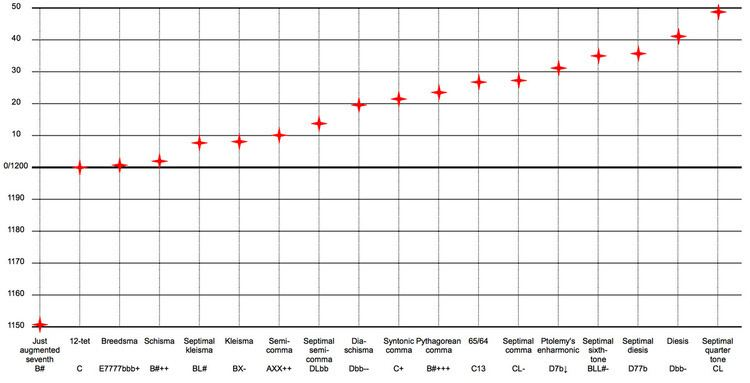# Enharmonic

Updated onIn modern musical notation and tuning, an enharmonic equivalent is a note, interval, or key signature that is equivalent to some other note, interval, or key signature but "spelled", or named differently. Thus, the enharmonic spelling of a written note, interval, or chord is an alternative way to write that note, interval, or chord. For example, in twelve-tone equal temperament (the currently predominant system of musical tuning in Western music), the notes C and D are enharmonic (or enharmonically equivalent) notes. Namely, they are the same key on a keyboard, and thus they are identical in pitch, although they have different names and different roles in harmony and chord progressions.

## Contents

In other words, if two notes have the same pitch but are represented by different letter names and accidentals, they are enharmonic. "Enharmonic intervals are intervals with the same sound that are spelled differently… [resulting], of course, from enharmonic tones."

Prior to this modern meaning, "enharmonic" referred to notes that were very close in pitch—closer than the smallest step of a diatonic scale—but not identical in pitch, such as F and a flattened note such as G. as in enharmonic scale. "Enharmonic equivalence is peculiar to post-tonal theory." "Much music since at least the 18th century, however, exploits enharmonic equivalence for purposes of modulation and this requires that enharmonic equivalents in fact be equivalent."

Some key signatures have an enharmonic equivalent that represents a scale identical in sound but spelled differently. The number of sharps and flats of two enharmonically equivalent keys sum to twelve. For example, the key of B major, with 5 sharps, is enharmonically equivalent to the key of C major with 7 flats, and 5 (sharps) + 7 (flats) = 12. Keys past 7 sharps or flats exist only theoretically and not in practice. The enharmonic keys are six pairs, three major and three minor: B major/C major, G minor/A minor, F major/G major, D minor/E minor, C major/D major and A minor/B minor. There are practically no works composed in keys that require double sharps or double flats in the key signature. In practice, musicians learn and practice 15 major and 15 minor keys, three more than 12 due to the enharmonic spellings.

For example the intervals of a minor sixth on C, on B, and an augmented fifth on C are all enharmonic intervals  Play . The most common enharmonic intervals are the augmented fourth and diminished fifth, or tritone, for example C–F = C–G.

Enharmonic equivalence is not to be confused with octave equivalence, nor are enharmonic intervals to be confused with inverted or compound intervals.

## Tuning enharmonics

In principle, the modern musical use of the word enharmonic to mean identical tones is correct only in equal temperament, where the octave is divided into 12 equal semitones. In other tuning systems, however, enharmonic associations can be perceived by listeners and exploited by composers.

## Pythagorean

In Pythagorean tuning, all pitches are generated from a series of justly tuned perfect fifths, each with a frequency ratio of 3 to 2. If the first note in the series is an A, the thirteenth note in the series, G is higher than the seventh octave (octave = ratio of 1 to 2, seven octaves is 1 to 27 = 128) of the A by a small interval called a Pythagorean comma. This interval is expressed mathematically as:

twelve fifths seven octaves = ( 3 2 ) 12 2 7 = 3 12 2 19 = 531 441 524 288 = 1.013 643 264... 23.46  cents

## Meantone

In quarter-comma meantone, on the other hand, consider G and A. Call middle C's frequency x. Then high C has a frequency of 2x. The quarter-comma meantone has just (i.e., perfectly-tuned) major thirds, which means major thirds with a frequency ratio of exactly 4 to 5.

To form a just major third with the C above it, A and high C must be in the ratio 4 to 5, so A needs to have the frequency

4 5 ( 2 x ) = 8 5 x = 1.6 x .

To form a just major third above E, however, G needs to form the ratio 5 to 4 with E, which, in turn, needs to form the ratio 5 to 4 with C. Thus the frequency of G is

( 5 4 ) 2 x = 25 16 x = 1.5625 x

Thus, G and A are not the same note; G is, in fact 41 cents lower in pitch (41% of a semitone, not quite a quarter of a tone). The difference is the interval called the enharmonic diesis, or a frequency ratio of 128/125. On a piano tuned in equal temperament, both G and A are played by striking the same key, so both have a frequency

2 8 12 x = 2 2 3 x 1.5874 x

Such small differences in pitch can escape notice when presented as melodic intervals. However, when they are sounded as chords, the difference between meantone intonation and equal-tempered intonation can be quite noticeable, even to untrained ears.

One can label enharmonically equivalent pitches with one and only one name; for instance, the numbers of integer notation, as used in serialism and musical set theory and employed by the MIDI interface.

## Enharmonic genus

In ancient Greek music the enharmonic was one of the three Greek genera in music in which the tetrachords are divided (descending) as a ditone plus two microtones. The ditone can be anywhere from 16/13 to 9/7 (3.55 to 4.35 semitones) and the microtones can be anything smaller than 1 semitone. Some examples of enharmonic genera are

1. 1/1 36/35 16/15 4/3
2. 1/1 28/27 16/15 4/3
3. 1/1 64/63 28/27 4/3
4. 1/1 49/48 28/27 4/3
5. 1/1 25/24 13/12 4/3

Topics